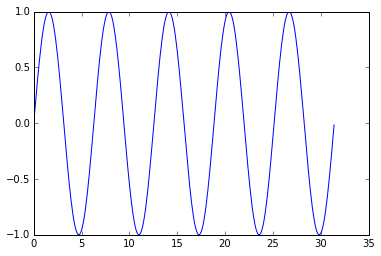# Introducing Jupyter (IPython Notebook)

Standard

In this entry, we are going to get to know one of the most useful Python tools ever created: Jupyter (formerly known as the IPython Notebook). Seriously, this thing is much more powerful than it sounds when first introduced: it is a Python interpreter that works with independent code and markdown cells in a browser.

Actually, since IPython Notebook became Jupyter, other languages are also supported apart from Python.

The great thing about Jupyter is that it lets us present code in a different way. Instead of executable files with comments, or a plain text/html/LaTex file with static code snippets, a Jupyter notebook contains separate text cells, that let us write extensive rich HTML documents with a subset of LaTex commands; and code cells with actual executable code and outputs in the same document.

Jupyter notebooks are especially good for presenting algorithms or explaining a piece of code. I personally use it when I am analyzing large datasets, since I can apply code over the data, see the results immediately and then try new stuff. Actually, I am writing this blog entry using Jupyter. Just how meta is that?

Jupyter is installed with the major Python commercial and free distributions, but not with the vanilla distribution. Anyway, it can be installed using pip:

pip install jupyter


To start it, we must start a terminal (cmd in Windows) and type:

ipython notebook


It will start the server and launch a browser with a file explorer:We can navigate through the file system and create new directories. To create a new notebook, we select New on the top right corner and select Python. This will open up a new tab with an untitled notebook. Lets dissect this screen:The first row contents the title of the notebook that can be modified and shows the status of the changes. The second row contains the menu bar that controls the cells and the interpreter. After that, a toolbar has some shortcuts. Here we can define if a cell is a code cell or markdown cell. So far, all this blog entry has been written in a markdown cell.

The code cells are numbered, and we can just write Python code and run it either by pressing the “play” icon or Caps+Enter in the keyboard (after some time, you will definitely use the keyboard).

In :
print("This is a Python code cell")
print("We can run any command and press Caps+Enter to run it")
print("The result will be displayed below the cell")

This is a Python code cell
We can run any command and press Caps+Enter to run it
The result will be displayed below the cell

In :
txt = """In a single notebook, all code cells are executed
in the same Python instance, so variables and imports work
across the cells """

In :
print(txt)

In a single notebook, all code cells are executed
in the same Python instance, so variables and imports work
across the cells


The interesting point of Jupyter is that it lets us do a visual and interactive approach to the problems. Interactive in the sense that if the results of a cell are not what we expected, we can modify it an re-run it without need of running again all the previous cells (unless we need to reset some variable). And visual since we can, for instance, plot data with matplotlib:

In :
%matplotlib inline
import matplotlib.pyplot as plt
import numpy as np

x = np.arange(0,10*np.pi,0.1)
y = np.sin(x)
plt.plot(x,y)
plt.show()The first line of this example is an IPython magic command, that modifies the behavior of the interpreter. In this case, we have indicated that we want the graph on the notebook, instead of in an external window. This is especially useful for presentations, but we can’t modify the plot or zoom.

The output is updated as the code is running, which is useful when the executed cell has some delay:

In :
from time import sleep

for i in range(5):
print(str(i)+' seconds')
sleep(1)

0 seconds
1 seconds
2 seconds
3 seconds
4 seconds


We can export the result to HTML, PDF or .py (a normal Python script where each markdown cell is translated to comments). Anyway, we can always return to the saved notebook and continue editing it and modifying code.

In this entry we have seen the main capabilities of this tool. In fact, it does not have too many options; it is a fairly simple program. But it opens a whole new dimension in the use of code, and that is what makes it a really powerful tool.

This site uses Akismet to reduce spam. Learn how your comment data is processed.